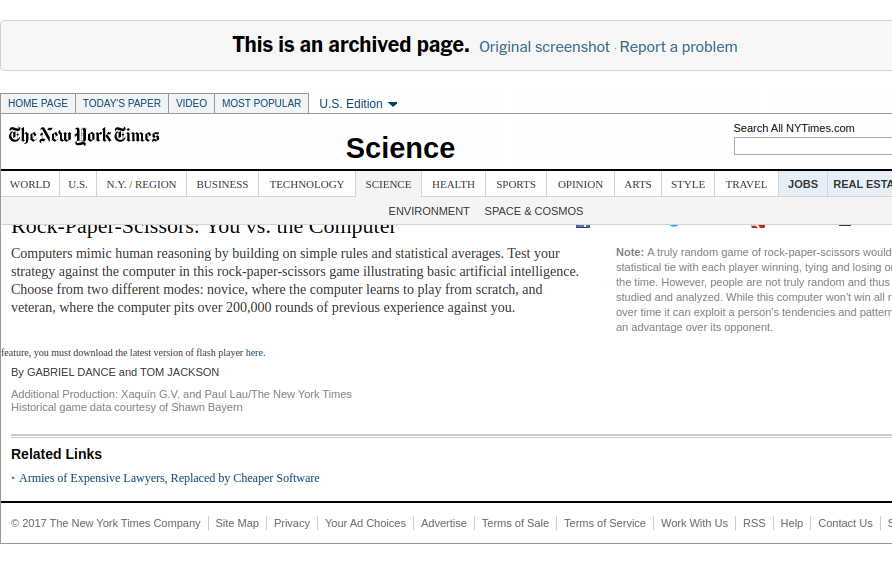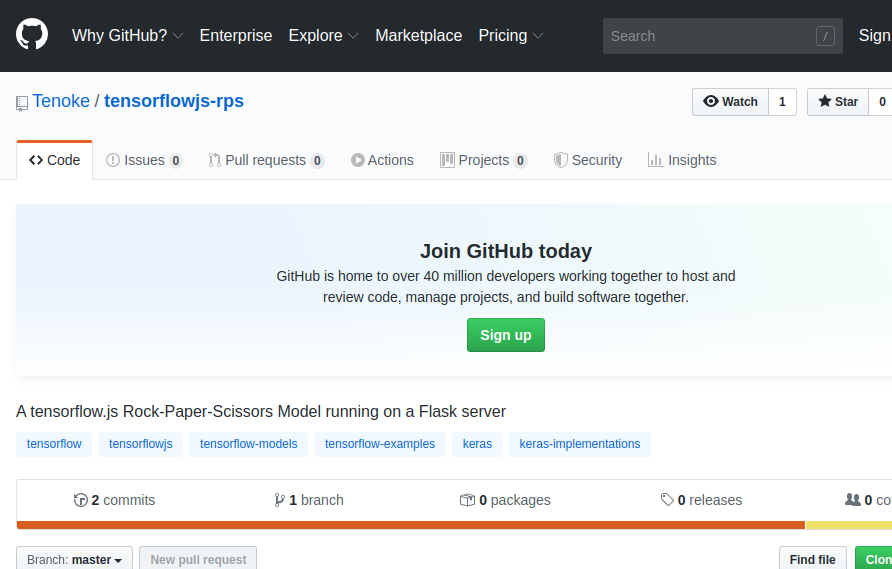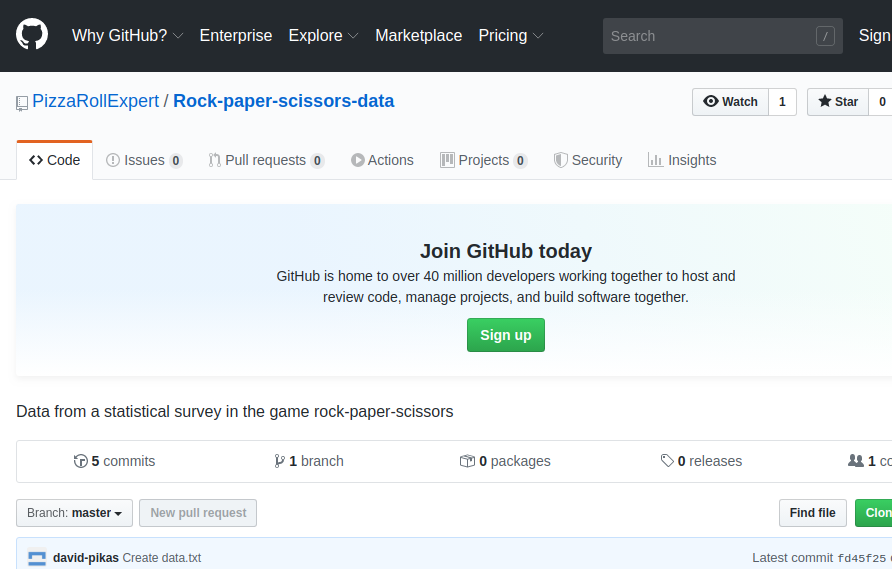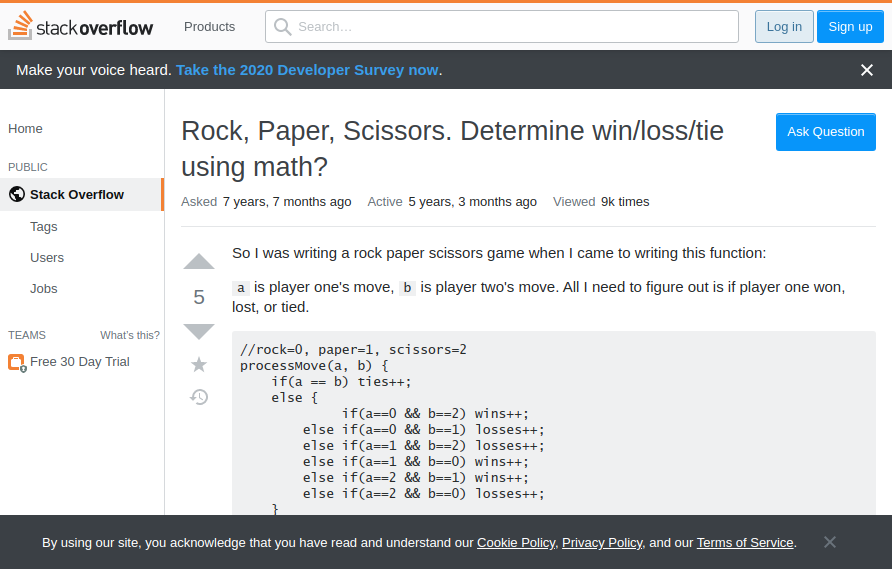As theNew York Times have demonstrated, an algorithm can consistently win against humans at Rock Paper Scissors (RPS) using the right statistical techniques. The fact that it is doable, the simplicity, and the popularity of the game make it a good choice for attempting to do it using Deep Learning and making it run with tensorflow.js.

The goals here are simple - we want to run the Neural Net in the user’s browser, and we want it to have some edge and win against most humans.

## Framing the problem

A common instinct when thinking of games is to have an agent-based approach using reinforcement learning - e.g. Q learning. However, reinforcement learning has a reputation for being somewhat finicky, unstable, and often relatively computationally expensive.

In this specific case, we can, however, frame the problem as a classification problem - predicting whether the next move by the human is going to be a Rock/Paper/Scissors and simply doing the opposite ourselves - which allows us to use somewhat more robust, standard and hopefully smaller models.

## Preparing to build the model

We are going to be running the finished model fully in the browser and despite it being possible to define everything in JavaScript, some things are easier to do in python. For one, the tensorflow.js API is not quite as complete and nice as the python one, and even more importantly - I find preprocessing of data much easier in python and working in a Jupyter notebook in general.

Thus, we are going to take advantage of being able to import a tensorflow/keras model into tensorflow.js, which as far as I can tell is almost always the better option.

Note: Theassociated repo has the code, data, and all pip-installable prerequisites.

### Overview of approach

We are going to use an LSTM, so data from the previous moves made by the player can influence a prediction of their next move, while also being able to work with a non-fixed number of moves. After we predict their next move, we are simply going to do the move which wins against them (e.g. if we predict they are going to throw rock, we throw paper).

We are also going to use a little trick to counter how weak the signal in the data is. Even though humans don’t quite play randomly (if they did, we wouldn’t ever be able to perform better against anyone, except by chance) they hardly follow an exceptionally easy to spot pattern either. In fact, we should expect it to be fairly hard, and data-intensive for our model to spot a pattern and to even start learning anything at all about the data thrown at it.

What we are going to do, in order to force the model to at least initially learn something about RPS is to give it a secondary objective - to guess who won the current round (for which it has all the data), and to keep track of how many wins it has against the player overall. A fairly easy objective, compared to our main objective of guessing the player’s next move.

This has two benefits - it makes the model learn something about RPS (mainly which hand wins against which other hand), and it is also extremely helpful while building the model - if we see that it is not even learning the secondary objective, then there is little hope for it to learn the main one. Indeed, this helped me identify multiple bugs during development.

### Imports

Let’s start by importing the main things we need - numpy and keras.

``````from tensorflow import keras
import numpy as np
``````

### Data

I looked for any RPS data to build the initial model on, and the first thing which popped out and seemed usable wasThis repo

``````%%bash
wget https://github.com/PizzaRollExpert/Rock-paper-scissors-data/raw/master/data.txt
``````

After downloading it we need to create a dict for converting the symbols in the dataset to numbers.

``````move_to_n = {
's':1,
'p':2,
'x':3
}
``````

We then create a function that iterates over all the moves in the files, converts them to numbers using the move_to_n dict, and splits all the moves that are part of the same game into arrays. The code can be simplified and sped up, but as it is for a one-time preprocessing of a very specifically formatted dataset, there’d be little point.

``````def convert_data(data):
result = []
game = []
for row in data:
moves = []
if row == '-':
if len(game) > 2:
result.append(np.array(game))
game = []
continue
for move in row:
moves.append(move_to_n[move])
if len(moves) > 1:
game.append(np.array(moves))
moves = []
return np.array(result)
``````

We then open the data we downloaded, split it by rows, convert it using our function, and then flip it - so we have the data both from the perspective of the first player (e.g. rock vs paper) and the second one (e.g. paper vs rock).

``````data = convert_data(data)
data2 = np.array([np.flip(games, 1) for games in data]) #reverse for player2
data = np.concatenate((data2, data))
``````

Now we have our X - the prepared data for passing to the model to base its guesses, but we need to create our y (ground truth), too.

First, we are going to make a small helper function for determining who won a given round. I googled around for an easier way to check who won instead of `if player1 == 'rock' and player2 ==..`, and I created the function below, based on what I sawHere. I admit, I had to triple-check if it works properly.

``````def calculate_winner(x):
result = x - x
if result == 0: return 0 #tie
if result in (1,-2): return 1 #win
return -1 #lose
``````

And then another function to create our matching X, and y. We go over each game (which has multiple moves by the same players), add a running score using `calculate_winner` for our secondary objective, and use next round’s move from player 1 as the portion of y in our primary objective.

``````def create_xy_winner(data):
result_y = []
result_x = []
for game in data:
game_y = []
score = 0
for i, moves in enumerate(game):
if i+1 == len(game):
result_y.append(np.array(game_y))
result_x.append(game[:-1]) #remove last moves from x
#skip last game as we dont know what player1 will choose next move
continue
score += calculate_winner(game[i])
game_y.append([game[i+1], score]) #append next player1 move
return np.array(result_x), np.array(result_y)

X, y = create_xy_winner(data)
``````

### Model

We have our data nicely formatted now, so it is time to create our model.

We start with an Input layer, which has dimensions (None, 8) - None for the timesteps (as many as we pass) which correspond to the rounds in a given game, and 8 because after playing with it, I realized that one-hot encoding our (e.g. Rock - Paper) input data works better after testing. We then add a Dense layer (Note: instead of one-hot encoding and Dense layer, we could’ve also just used an Embedding). After that is our LSTM layer, which is the key to remembering and using information from previous rounds. We then add two Dense layers in a row, with the second one outputting a probability representing whether we expect player 1 to throw, rock paper, or scissors. We then do the same for our secondary objective - how many wins has player 1 had so far, which is a single number.

``````main_input = keras.Input(shape=(None,8), dtype='float32')
dense = keras.layers.Dense(32, activation='relu')(main_input)
lstm = keras.layers.LSTM(96, return_sequences=True)(dense)
main_output = keras.layers.Dense(20, activation='relu')(lstm)
main_output = keras.layers.Dense(4, activation='softmax')(main_output)
second_output = keras.layers.Dense(20, activation='tanh')(lstm)
second_output = keras.layers.Dense(1)(second_output)
model = keras.Model(inputs=[main_input,], outputs=[main_output, second_output])
``````

We also create an optimizer - Adam with the normal defaults, and compile the model, so the primary objective is treated as much more important (1.0) than our secondary objective (0.2), and we choose their respective loss functions - categorical crossentropy for the primary (as we are choosing categories - rock, paper or scissors), and mean squared error for the number of wins.

``````opt = keras.optimizers.Adam(lr=0.001)
model.compile(loss=['categorical_crossentropy', 'mse'], optimizer=opt, metrics=['accuracy'], loss_weights=[1., 0.2])
``````

That’s it for the model. I also attempted some variations - adding Dropout, Batchnorm, and more/less layers, but the current implementation worked best out of those I tried.

### Training

Now, is the time to train our model, however, I get an error when I try to model.fit the data - The model expects our data to be one-hot encoded (or more specifically to have a shape of *,8 rather than *,2), but I didn’t do that initially. Now, because we are working with so little data, and a tiny model (all of it trains in a few seconds on my CPU), we can just do it as we pass the data.

We iterate over all the games we have, one-hot encode our X and primary y, using the built-in keras function `to_categorical`, re-shape the data until we get it how the model expects it (I rarely get this quite right on the first try) and train the model using a batch size of 1.

``````    for i in range(len(X)):
X_, y_ = X[i], y[i]
X_ = keras.utils.to_categorical(X_, 4)
X_ = X_.reshape(1, X_.shape, 8)
y_1 = keras.utils.to_categorical(y_[:,0].reshape(1, y_.shape, 1), 4)
y_2 = y_[:,1].reshape(1, y_.shape, 1)
verbose = 2 if (i % 15 == 0) else 0 # print only 1/25th of the time
model.fit(X_, [y_1, y_2], epochs=1, shuffle=False, batch_size=1, verbose=verbose)
``````

Now, we have a trained model and it seems to reach an accuracy of 1 for guessing the number of wins (after fixing a couple of bugs), so we know the model is doing something right. After testing it out though, it will sometimes be very exploitable (e.g. getting stuck into throwing scissors 10 times in a row).

So, my next step was to increase the data we have - mainly by playing a bunch of games against it and then re-training by adding the new data in. After doing that, I sent it to 12 people, with only one of them managing to win (after at least 50 rounds), so I added their data to main data and re-trained it again.

Realistically, the model is somewhat overfitted against playing against me, as that is where the bulk of the data comes from - and it really does wipe the floor with me. If we truly want to optimize the model, we’d keep collecting more data from different people and keep training it, but it already seems to be able to win against most people (given a sufficient number of rounds) which is good enough for our purposes.

### Exporting the model

Now that we have built and trained our model we just need to load it with tensorflow.js and make it work in the browser. We first export it.

``````import tensorflowjs as tfjs
tfjs.converters.save_keras_model(model, 'Full-Model')
``````
``````%%bash
rm -rf static/Full-Model
mv Full-Model static/
``````

### Importing the model to JavaScript

All we need in order to load our model is to import tensorflow.js, which we will do in our html via a CDN.

``````<script src="https://cdn.jsdelivr.net/npm/@tensorflow/tfjs@0.11.2"> </script>
``````

After that, we can simply load it in our JavaScript like so

``````const fullModel  = tf.loadModel('/static/Full-Model/model.json')
``````

Note: A lot of things are still broken in tensorflow.js - for one, if you name your layers yourself, you can’t import the model. For another, it doesn’t support the default naming of the newest version of tensorflow either. If you have any problems, try using an older version of tensorflow, or tensorflow.js. When I had that issue, it was most easily fixed using `import keras` instead of `from tensorflow import keras`, with newest versions from pip/conda for both.

After we have loaded the model we create the dicts for converting from number to move, and back.

``````n_to_move = {
1:'rock',
2:'paper',
3:'scissors'
}

move_to_n = {
'rock': 1,
'paper': 2,
'scissors': 3
}
``````

We also choose a random first move (as we have no data yet) and create a list to hold the moves so far and some counters for the wins.

``````function getRandomInt(max, starting=1) {
return (starting + Math.floor(Math.random() * max))
}
//Choose a random first move
let nextComputerMove = getRandomInt(3)
currentMoves = []
let humanWinCounter = 0
let computerWinCounter = 0
let tieCounter = 0
``````

We then create a copy of the `to_categorical` function we used for one-hot encoding the data, as it doesn’t exist in tensorflow.js, and a function to determine who has won (and update the win counters)

``````function to_categorical(n, length=4) {
let result = Array.from({length}, ()=> 0.)
result[n] = 1.
return result
}

function updateCounters(humanMove, computerMove) {
switch (humanMove - computerMove) {
case 0:
tieCounter++
return 0
case  1:
case -2:
humanWinCounter++
return 1
default:
computerWinCounter++
return 2
}
}
``````

We will also need a function to determine our move based on our prediction of the player’s next move (e.g. if we predict rock, throw paper)

``````
function moveBasedOn(move) {
switch(move) {
case 1:
return 2
case 2:
return 3
case 3:
return 1
}
}
``````

Now we can create a function, which uses the moves done by the player and computer so far, and uses the model to choose our next move. We only use the last 28 moves - I tried a few numbers between the last 20 and last 35 moves, and somewhere between 25 and 30 seemed to perform best in my limited testing, likely due to the nature of our training data in combination with LSTMs sometimes getting more brittle after a larger number of timesteps.

Tensorflow.js functions are mostly asynchronous (with some of them having a synchronous version like .dataSync), so any function which works with the model needs to be asynchronous, too.

``````async function calculateNextComputerMove() {
const model = await fullModel
let lastMoves = currentMoves.slice(Math.max(currentMoves.length-28, 0), currentMoves.length)
let data = tf.tensor3d([lastMoves], [1, lastMoves.length, 8])
let nextHumanMovePrediction = model.predict(data).as1D()
// we are getting predictions for all moves (because of how we trained the network)
// but only care for the prediction for the next move - the last 4 numbers
nextHumanMovePrediction = nextHumanMovePrediction.slice(nextHumanMovePrediction.shape-4,4)
// next we turn that into a single number from a one-hot encoding
nextHumanMovePrediction = nextHumanMovePrediction.argMax().dataSync()
// and turn that into our next move based on what will beat the human
return moveBasedOn(nextHumanMovePrediction)
}
``````

When we have that, all that is needed is a function that takes the player’s move, shows them the model’s move (which has been calculated before they even made theirs), updates the counters and uses the current data to calculate the model’s next move.

``````function chooseMove(move) {
console.log('human: ', n_to_move[move])
var winner = updateCounters(move, nextComputerMove)
console.log(winner) # 0 = tie, 1 = human, 2 = computer
currentMoves.push(to_categorical(move).concat(to_categorical(nextComputerMove)))
calculateNextComputerMove().then(nextMove=>nextComputerMove=nextMove)
}
``````

That’s it. You can now play against the model by opening the console in your browser, and calling chooseMove(..) with ‘rock’, ‘paper’ or ‘scissors’, or building a frontend around the model like the one in thegithub repo accompanying this post.